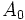# Non-normal subgroups of dihedral group:D8

(diff) ← Older revision | Latest revision (diff) | Newer revision → (diff)
This article is about a particular subgroup in a group, up to equivalence of subgroups (i.e., an isomorphism of groups that induces the corresponding isomorphism of subgroups). The subgroup is (up to isomorphism) cyclic group:Z2 and the group is (up to isomorphism) dihedral group:D8 (see subgroup structure of dihedral group:D8).
VIEW: Group-subgroup pairs with the same subgroup part | Group-subgroup pairs with the same group part | All pages on particular subgroups in groups

## Definition

Suppose$G$ is the dihedral group of order eight (degree four) given by the presentation:$G := \langle a,x \mid a^4 = x^2 = e, xax = a^{-1} \rangle$.

Then, we are interested in the following four subgroups:$A_0 := \langle x \rangle = \{ x, e \}, A_1 := \langle ax \rangle = \{ ax, e \}, A_2 := \langle a^2x \rangle = \{ a^2x, e \}, A_3 = \langle a^3x \rangle = \{ a^3x, e \}$.$A_0$ and$A_2$ are conjugate subgroups (via$a$, for instance).$A_1$ and$A_3$ are conjugate subgroups (via$a$, for instance).$A_0$ and$A_1$ are not conjugate but are related by an outer automorphism that fixes$a$ and sends$x$ to$ax$. Thus, all four subgroups are automorphic subgroups. These are the only non-normal subgroups of$G$ and they are all 2-subnormal subgroups.

## Arithmetic functions

Function Value Explanation
order of whole group 8
order of subgroup 2
index 2
size of conjugacy class 2
number of conjugacy classes in automorphism class 2
size of automorphism class 2

## Effect of subgroup operators

Specific values (in the second column) are for$A_0 = \langle x \rangle$.

Function Value as subgroup (descriptive) Value as subgroup (link) Value as group
normalizer$\langle a^2, x \rangle$ Klein four-subgroups of dihedral group:D8 Klein four-group
centralizer$\langle a^2,x \rangle$ Klein four-subgroups of dihedral group:D8 Klein four-group
normal core$\{ e \}$ -- trivial group
normal closure$\langle a^2,x \rangle$ Kein four-subgroups of dihedral group:D8 Klein four-group
characteristic core$\{ e \}$ -- trivial group
characteristic closure$G$, i.e.,$\langle a,x \rangle$ -- dihedral group:D8

## Related subgroups

### Intermediate subgroups

We use$A_0 = \langle x\rangle$ here.

Value of intermediate subgroup (descriptive) Isomorphism class of intermediate subgroup Small subgroup in intermediate subgroup Intermediate subgroup in big group$\langle a^2, x \rangle$ Klein four-group Z2 in V4 Klein four-subgroups of dihedral group:D8